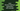# Java Stream findAny method explanation with example### Java Stream findAny method explanation with example:

findAny is used to get any element of a Java stream. If the stream is empty, it returns one empty optional. The return value is of type Optional. If the element selected is null, it throws one NullPointerException.

In this post, we will see different examples of findAny and how it works. Note that it is a nondeterministic operation and it can return any element from the stream.

### Syntax of findAny:

findAny is defined as:

``Optional<T> findAny()``
• So, the return value is contained in an Optional. It may contain a null or non-null value. For null element selection, it throws NullPointerException.

### Example to get any element from a list of integer stream using findAny:

Let’s take a look at the below example program:

``````package com.company;

import java.util.Arrays;
import java.util.Optional;
import java.util.stream.Stream;

public class Main {
public static void main(String[] args){
Stream<Integer> resultStream = Arrays.asList(1,2,3,4,5,6,7,8,9).stream();

Optional value = resultStream.findAny();

if(value.isPresent()){
System.out.println(value.get());
}
}
}``````

Output:

``1``

It is a nondeterministic result. If we do multiple iterations on the same data source, it might give different result.

### Example of findAny with a list of strings:

Let’s try to use findAny with a list of strings:

``````package com.company;

import java.util.Arrays;
import java.util.Optional;
import java.util.stream.Stream;

public class Main {
public static void main(String[] args){
Stream<String> resultStream = Arrays.asList("Sun", "Mon", "Tue", "Wed", "Thurs", "Fri", "Sat").stream();

Optional value = resultStream.findAny();

if(value.isPresent()){
System.out.println(value.get());
}
}
}``````

It is similar to the above program. The only difference is that we are using a stream of strings instead of a stream of integers.

It will print the below output:

``Sun``

### Using with a parallel stream:

We can also use it with a parallel stream:

``````package com.company;

import java.util.Arrays;
import java.util.Optional;
import java.util.stream.Stream;

public class Main {
public static void main(String[] args){
Stream<String> resultStream = Arrays.asList("Sun", "Mon", "Tue", "Wed", "Thurs", "Fri", "Sat").parallelStream();

Optional value = resultStream.findAny();

if(value.isPresent()){
System.out.println(value.get());
}
}
}``````

It will print:

``Thurs``

Adding a filter works similar to filter with stream. For example:

``````import java.util.Arrays;
import java.util.Optional;
import java.util.stream.Stream;

public class Main {
public static void main(String[] args){
Stream<Integer> resultStream = Arrays.asList(1,2,3,4,5,6,7,8,9,10).stream();

Optional value = resultStream.filter(x -> x%2 == 0).findAny();

if(value.isPresent()){
System.out.println(value.get());
}
}
}``````

Here, we are using filter to filter out all the even numbers from the integer stream. It will print 2 as the output.